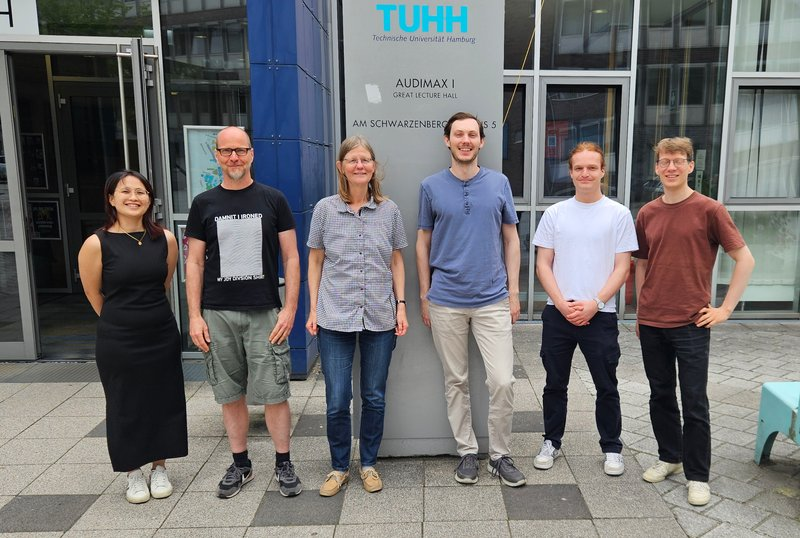TUHH / Institut für Mathematik / Lehrstuhl Numerische Mathematik# Lehrstuhl Numerische MathematikProf. Dr. Sabine Le Borne
Prof. Dr. Wolfgang Mackens (i. R.)
Prof. Dr. Heinrich Voß (i. R.)
Technische Universität Hamburg
Institut für Mathematik (E-10)

Am Schwarzenberg-Campus 3
21073 Hamburg

Sekretariat:
Margitta Janssen
Raum 3.048
Tel: +49 40 42878-3278
E-Mail: m.janssen@tuhh.de

## Forschungsgebiete

#### Prof. Dr. Sabine Le Borne

• Iterative methods and preconditioners for large sparse linear systems of equations

The direct solution of a linear system of equations $Ax=b$, the Gauß eliminiation, becomes prohibitively expensive as theproblem size $n$ increases. Several iterative methods have been developed that compute an approximation $\tilde{x}$ to the exact solution $x$. The objective is to obtain such an approximation using computational and storage complexities which are linear in the problem size $n$.

Several iterative methods can be accelerated through so-called preconditioners. Here, one can distinguish between blackboxand problem-dependent techniques. Blackbox techniques require only the problem matrix $A$ and right hand side vector $b$ as input. This is typically very convenient for the user since no additional knowledge on the underlying problem or the preconditioner itself is required, but also leads to slower methods in applications where problem-dependent information is available and successfully exploited in the design of the preconditioner

We are interested in various aspects of the development of efficient preconditioning techniques, typically taylored to specific applications and discretization techniques. As a model problem, we consider the discretization of partial differential equations (pde). Both the underlying pde as well as the discretization technique determine the structure, and condition number, of the resulting linear system of equations. For the case of convection-dominated problems, we have developed ordering techniques which reorder the underlying unknowns prior to the iterative solution, yielding significant improvements already when used in combination with rather standard preconditioners. Mixed finite elements lead to a characteristic block structure in the matrix, and we have developed several block preconditioners exploiting such a structure. Similar ideas can be applied to matrices which result from using hierarchical bases in the underlying finite element spaces.Figure 1: Typical sparsity plots - matrix resulting from a fourth order FE discretization of the Laplace problem (left), and the matrix after a nested dissection row and column reordering.

• Hierarchical matrices - theory and applications

Hierarchical ($\mathcal {H}$-) matrices provide a technique for the sparse approximation and matrix arithmetic of large, fully populated matrices. The two main ingredients of a successful $\mathcal {H}$-matrix construction are

• a (hierarchical) block partition of the matrix which includes a reordering of the unknowns;

• low-rank approximations of the matrix data within these blocks.

In the case of sparse matrices, $\mathcal {H}$-matrices and their arithmetic become interesting for the computation of (approximate) matrix inverses, LU, QR, or other decompositions which are no longer sparse. While the $\mathcal {H}$ arithmetic has reached a relatively mature state, it is the construction of the hierarchical block partition which is of current research interest. Typically, a domain-decomposition based block partitioning turns out to be superior (wrt computational effort required to reach a certain accuracy in the subsequent matrix factorization) to bisection-based partitionings for sparse matrices. In general, the underlying (class of) applications (or the desired matrix operation) determine the block partitioning suitable for efficient low-rank blockwise approximations (given its existence, which is not always granted).Figure 2: Typical $\mathcal {H}$-matrix block structures for a discretized Laplace problem in 3d, bisection based (left) and domain-decomposition (right) clustering.}

• Numerical methods in Computational Fluid Dynamics

The core system of (partial differential) equations modeling fluid flow is provided by the Navier-Stokes equations. Its numerical treatment involves several numerical challenges, including time and space discretizations as well as the need for efficient nonlinear and linear solvers. Our research focus lies in the development and analysis of efficient solution techniques for the underlying linear systems of equations which consist of a sequence of (hard to solve) saddle point problems of the form

$$\left( \begin{array}{cc} A^{{(k)}} & B \\ B^T & 0 \end{array} \right) \left( \begin{array}{c} u^{{(k+1)}} \\ p^{{(k+1)}} \end{array} \right) = \left( \begin{array}{c} f^{{(k)}} \\ g^{{(k)}} \end{array} \right).$$

Recent years have seen much work and progress on the efficient iterative solution of these types of linear systems. A popular - and to date the most promising - approach is based on an approximate block LU factorization which requires the solution of a Schur complement problem as a subproblem. The development of efficient preconditioning techniques for such Schur complements, especially for those resulting from flow simulations in three spatial dimensions, is an active field of research.

• Scattered data interpolation with radial basis functions

Text folgt

• Numerical methods for population balance equations/multivariate particle systems

Text folgt

#### Dr. Jens Zemke

• Untersuchung der numerischen Stabilität von Krylov-Unterraum-Verfahren

Die verstärkt auftretenden großen, dünnbesetzten Gleichungssysteme werden aus Speicher- und Rechenzeitgründen immer häufiger mittels iterativer Verfahren gelöst. Eine wichtige Klasse dieser iterativen Verfahren ist die der Krylov-Unterraum-Verfahren. Diese sind der Theorie nach direkte Verfahren, d.h. sie lösen das Gleichungssystem in endlich vielen Schritten exakt. In endlicher Arithmetik ergeben sich eine Reihe von mehr oder minder starken Abweichungen, insbesondere in Bezug auf den Verlust der Orthogonalität der konstruierten Basis und der Konvergenzgeschwindigkeit. Diese Abweichungen sind am stärksten im Falle sogenannter Kurz-Term-Rekursionen, e.g., CG und Lanczos, welche gleichzeitig die Klasse der effektivsten Verfahren im Hinblick auf Speicherbedarf und Rechenzeit pro Schritt bilden.

Die bisherigen Analysen des Verhaltens von Krylov-Unterraum-Verfahren in endlicher Genauigkeit sind nicht hinreichend um alle beobachtbaren Phänomene tatsächlich zu erklären. Ein Grund für das Scheitern der bisherigen Analysen scheint im Festhalten an den Termini Rückwärtsfehler, Vorwärtsfehler und gemischter Vorwärt- Rückwärtsfehler, wie sie bei dichtbesetzten Gleichungssystemen Anwendung finden, zu liegen. Hier ist Raum für eine Verbesserung sowohl der Analysen als auch der Begrifflichkeiten.